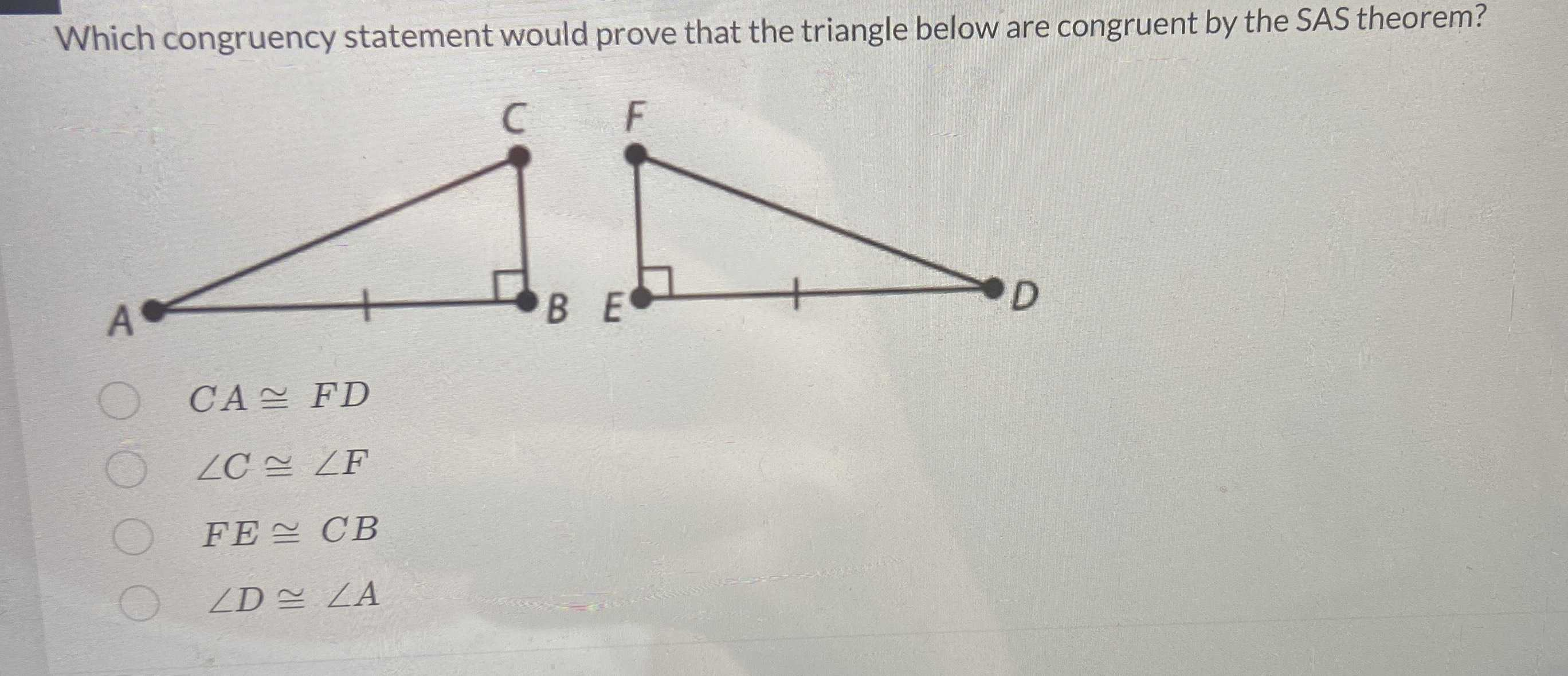### Still have math questions?

Trigonometry
QuestionWhich congruency statement would prove that the triangle below are congruent by the SAS theorem?

A. $$C A \cong F D$$

B. $$\angle C \cong \angle F$$

C. $$F E \cong C B$$

D. $$\angle D \cong \angle A$$